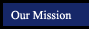Geometry Postulates and Theorems
textbook tutorials - definitions - constructions - postulates and theorems - internet activities and resources
 symmetry and similarity points, lines and planes parallel lines triangles quadrilaterals polygons circles 3-Dimensional Geometry
If two parallel lines are intersected by a transversal
then alternate interior angles are congruent.
In a plane if two lines are both perpendicualar to a third line,
then the two lines are parallel.
If two parallel lines are intersected by a transversal
then same-side interior angles are supplementary.
If two lines are both parallel to a third line,
then the two lines are parallel.
If two lines are intersected by a tranversal and alternate interior angles are congruent,
then the lines are parallel.
If two parallel lines are intersected by a third line,
then the lines of intersection are parallel.
If two lines are intersected by a tranversal and same-side interior angles are supplementary
then the lines are parallel.
If two planes are both parallel to a third plane,
then the two planes are parallel.